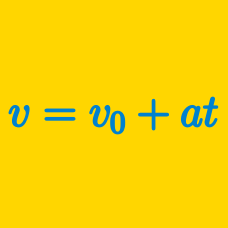Classical Mechanics

# Velocity Vector for Constant Acceleration

An object is thrown straight down with an initial speed of $5\text{ m/s}$ from a bridge. If the atmosphere is completely free of air resistance, how fast will the stone be when it enters the water $0.5$ seconds later?

The gravitational acceleration is $g=10\text{ m/s}^2.$

An object is thrown straight upward with an initial speed of $31\text{ m/s}.$ If the atmosphere is completely free of air resistance, what is its velocity after $4$ seconds has passed?

Define upward as positive direction.
The gravitational acceleration is $g=10\text{ m/s}^2.$

A bullet is being fired from a pistol. As the bullet is fired, it initially accelerates uniformly at $1000\text{ m/s}^2$ for $0.15$ seconds. After $0.15$ seconds has passed and the bullet has escaped from the pistol, it constantly decelerates at a rate of $1\text{ m/s}^2$ due to air resistance. What is the speed of the bullet $4.15$ seconds after the trigger is pulled?

Ignore the effect of gravity.

An object is falling with a speed of $3\text{ m/s}.$ If the atmosphere is free of air resistance, how fast will the object be falling $3$ seconds later?

The gravitational acceleration is $g=10\text{ m/s}^2.$

A car that is initially traveling at $19\text{ m/s}$ accelerates uniformly for $5.0$ seconds at a rate of $4.0\text{ m/s}^2,$ in a straight line. What is the car's velocity after the $5.0$ seconds?

×